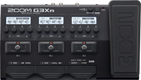# Banbeg

Discussion in 'Zoom G3n/G3Xn/G5n' started by Banbeg, Dec 31, 2022.

1. BanbegDevice: Zoom G3Xn
Firmware: 2.20

Name on device: Highway St
Optimized for: Phones/Speaker

Effects chain:Blackmore ish

Effect: "GoldDrive" (Overdrive / Distortion), active - "yes"
"Gain" = 32
"Bass" = 81
"Treble" = 29
"Volume" = 41

Effect: "MS 1959" (Amp simulator), active - "yes"
"Bass" = 84
"Middle" = 18
"Treble" = 31
"Presence" = 0
"Input1" = 42
"Input2" = OFF
"Volume" = 60
"SOLO" = 1

Effect: "ZNR" (Dynamics), active - "yes"
"Detect" = EFXIN
"Depth" = 100
"Threshold" = 93
"Decay" = 0

Effect: "Gt GEQ" (Filter), active - "yes"
"160 Hz" = 4.0
"400 Hz" = 3.0
"800 Hz" = 1.0
"3.2 kHz" = 3.0
"6.4 kHz" = 0.0
"12.0 kHz" = 0.0
"Volume" = 35
"CH SEL" = UnLATCH
"160 Hz" = 0.0
"400 Hz" = 0.0
"800 Hz" = 0.0
"3.2 kHz" = 0.0
"6.4 kHz" = 0.0
"12.0 kHz" = 12.0
"Volume" = 0
"CH SEL" = LATCH
"Parameter set" = A

Effect: "EarlyRef" (Reverb), active - "yes"
"Decay" = 15
"Shape" = 10
"Tone" = 6
"Mix" = 50

Patch Volume: 100